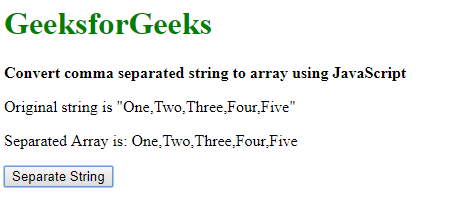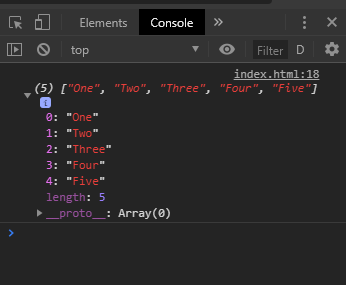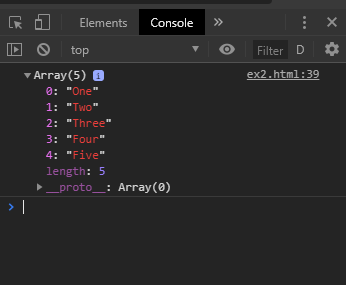# Convert comma separated string to array using JavaScript

• Last Updated : 23 May, 2019

A comma-separated string can be converted to an array by 2 approaches:

Method 1: Using split() method
The split() method is used to split a string on the basis of a separator. This separator could be defined as a comma to separate the string whenever a comma is encountered. This method returns an array of strings that are separated.

Hey geek! The constant emerging technologies in the world of web development always keeps the excitement for this subject through the roof. But before you tackle the big projects, we suggest you start by learning the basics. Kickstart your web development journey by learning JS concepts with our JavaScript Course. Now at it's lowest price ever!

Syntax:

`string.split(', ')`

Example:

 ```<``html``>`` ` `<``head``>``    ``<``title``>``      ``Convert comma separated ``      ``string to array using JavaScript``  `````` ` `<``body``>``    ``<``h1` `style``=``"color: green"``>``      ``GeeksforGeeks``  ````    ``<``b``>Convert comma separated string ``      ``to array using JavaScript``    ``<``p``>Original string is``      ``"One, Two, Three, Four, Five"``    ``<``p``>``      ``Separated Array is: <``span` `class``=``"output"``>``  ```` ` `    ``<``button` `onclick``=``"separateString()"``>``      ``Remove Text``  ````    ``<``script` `type``=``"text/javascript"``>``        ``function separateString() {``            ``originalString = "One, Two, Three, Four, Five";``            ``separatedArray = originalString.split(', ');`` ` `            ``console.log(separatedArray);``            ``document.querySelector('.output').textContent = ``              ``separatedArray;``        ``}``    `````` ` ``

Output:

• After clicking the button:• Console Output:Method 2: Iterating through the array keeping track of any comma encountered and creating a new array with the separated strings.
This approach involves iterating through each character in the string and checking for the comma. A variable previousIndex is defined which keeps the track of the first character of the next string. The slice method is then used to remove the portion of the string between the previous index and the current location of the comma found. This string is then pushed on to a new array. This process is then repeated for the whole length of the string. The final array contains all the separated strings.

Syntax:

 `originalString = ``"One, Two, Three, Four, Five"``;``separatedArray = [];` `// index of end of the last string ``let previousIndex = 0;` `for``(i = 0; i < originalString.length; i++) {` `    ``// check the character for a comma``    ``if` `(originalString[i] == ``', '``) {` `        ``// split the string from the last index``        ``// to the comma``        ``separated = originalString.slice(previousIndex, i);``        ``separatedArray.push(separated);` `        ``// update the index of the last string``        ``previousIndex = i + 1;``    ``}``}` `// push the last string into the array``separatedArray.push(originalString.slice(previousIndex, i));`

Example:

 ```<``html``>`` ` `<``head``>``    ``<``title``>``      ``Convert comma separated string``      ``to array using JavaScript``  `````` ` `<``body``>``    ``<``h1` `style``=``"color: green"``>``      ``GeeksforGeeks``  ````    ``<``b``>Convert comma separated ``      ``string to array using JavaScript``    ``<``p``>Original string is``      ``"One, Two, Three, Four, Five"``    ``<``p``>``      ``Separated Array is: <``span` `class``=``"output"``>``  ```` ` `    ``<``button` `onclick``=``"separateString()"``>``      ``Remove Text``  ````    ``<``script` `type``=``"text/javascript"``>``       ` `        ``function separateString() {``           ` `            ``originalString =``              ``"One, Two, Three, Four, Five";``            ``separatedArray = [];`` ` `            ``// index of end of the last string ``            ``let previousIndex = 0;`` ` `            ``for (i = 0; i < ``originalString.length``; i++) {`` ` `                ``// check the character for a comma``                ``if (originalString[i] == ', ') {`` ` `                    ``// split the string from the last index``                    ``// to the comma``                    ``separated` `= ``                      ``originalString``.slice(previousIndex, i);``                    ``separatedArray.push(separated);`` ` `                    ``// update the index of the last string``                    ``previousIndex` `= i + 1;``                ``}``            ``}`` ` `            ``// push the last string into the array``            ``separatedArray.push(``              ``originalString.slice(previousIndex, i));`` ` `            ``console.log(separatedArray);``            ``document.querySelector(``              ``'.output')``.textContent` `= ``separatedArray``;``        ``}``    `````` ` ``

Output:

• After clicking the button:• Console Output:My Personal Notes arrow_drop_up